# HashMap(JDK1.8)源码解析

## 简介

### 特点

HashMap 可以说是我们使用最多的 Map 集合，它有以下特点：

• 键不可重复，值可以重复
• 底层哈希表
• 线程不安全
• 允许key为null，value也可以为null

### JDK1.8之前

JDK1.8之前采用的是拉链法。拉链法：将链表和数组相结合。也就是说创建一个链表数组，数组中每一格就是一个链表。若遇到哈希冲突，则将冲突的值加到链表中即可。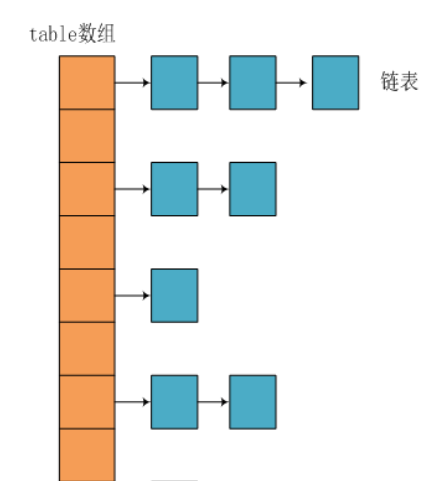### JDK1.8之后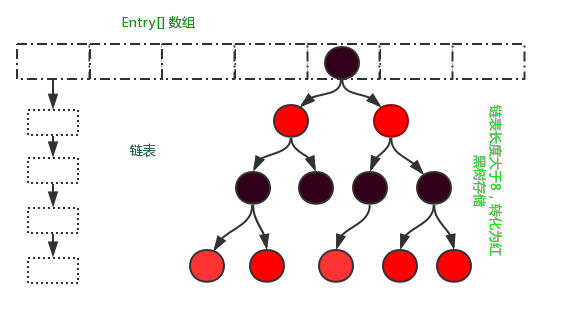### JDK1.7 VS JDK1.8 比较

JDK1.8主要解决或优化了一下问题：

1. resize 扩容优化
2. 引入了红黑树，目的是避免单条链表过长而影响查询效率，红黑树算法请参考
3. 解决了多线程死循环问题，但仍是非线程安全的，多线程时可能会造成数据丢失问题。

hash值计算方式扰动处理 = 9次扰动 = 4次位运算 + 5次异或运算扰动处理 = 2次扰动 = 1次位运算 + 1次异或运算

## 继承关系图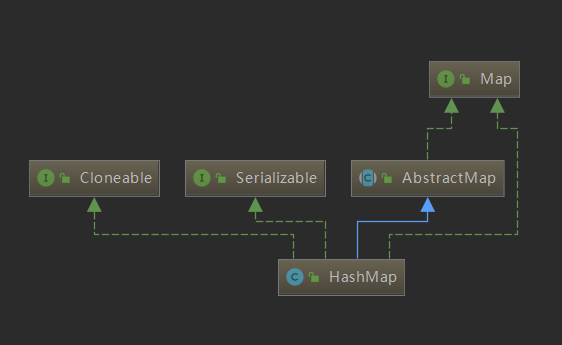HashMap继承抽象类AbstractMap，实现Map接口。除此之外，它还实现了两个标识型接口，这两个接口都没有任何方法，仅作为标识表示实现类具备某项功能。Cloneable表示实现类支持克隆，java.io.Serializable则表示支持序列化。

## 成员变量

//默认初始化Node数组容量16
static final int DEFAULT_INITIAL_CAPACITY = 1 << 4;
//最大的数组容量
static final int MAXIMUM_CAPACITY = 1 << 30;
//默认负载因子0.75
static final float DEFAULT_LOAD_FACTOR = 0.75f;
//由链表转红黑树的临界值
static final int TREEIFY_THRESHOLD = 8;
//由红黑树转链表的临界值
static final int UNTREEIFY_THRESHOLD = 6;
//桶转化为树形结构的最小容量
static final int MIN_TREEIFY_CAPACITY = 64;
//HashMap结构修改的次数，结构修改是指更改HashMap中的映射数或以其他方式修改其内部结构(例如，rehash的修改)。该字段用于在Collection-views上快速生成迭代器。
transient int modCount;
//Node数组下一次扩容的临界值，第一次为16*0.75=12（容量*负载因子）
int threshold;
//负载因子
//map中包含的键值对的数量
transient int size;
//表数据，即Node键值对数组，Node是单向链表，它实现了Map.Entry接口，总是2的幂次倍
transient Node<K,V>[] table;
//存放具体元素的集,可用于遍历map集合
transient Set<Map.Entry<K,V>> entrySet;


• capacity table的容量，默认容量是16
• threshold table扩容的临界值

## 构造方法

//初始化容量以及负载因子
public HashMap(int initialCapacity, float loadFactor) {
//判断初始化数组的容量大小
if (initialCapacity < 0)
throw new IllegalArgumentException("Illegal initial capacity: " +
initialCapacity);
if (initialCapacity > MAXIMUM_CAPACITY)
initialCapacity = MAXIMUM_CAPACITY;
//判断初始化的负载因子大小和是否为浮点型
throw new IllegalArgumentException("Illegal load factor: " +
//初始化负载因子
this.threshold = tableSizeFor(initialCapacity);
}

//初始化容量
public HashMap(int initialCapacity) {
}

//默认构造方法
public HashMap() {
}

//把另一个Map的值映射到当前新的Map中
public HashMap(Map<? extends K, ? extends V> m) {
putMapEntries(m, false);
}


• 定义初始容量大小（table数组的大小，缺省值为16），定义负载因子（缺省值为0.75）的形式

• 直接拷贝别的HashMap的形式，在此不作讨论

tableSizeFor()方法说明

//HashMap 中 table 角标计算及table.length 始终为2的幂，即 2 ^ n
//返回大于initialCapacity的最小的二次幂数值
static final int tableSizeFor(int cap) {
int n = cap - 1;
n |= n >>> 1;
n |= n >>> 2;
n |= n >>> 4;
n |= n >>> 8;
n |= n >>> 16;
return (n < 0) ? 1 : (n >= MAXIMUM_CAPACITY) ? MAXIMUM_CAPACITY : n + 1;
}


## 静态内部类

### Node

HashMap将hash，key，value，next已经封装到一个静态内部类Node上。它实现了Map.Entry<K,V>接口。

static class Node<K,V> implements Map.Entry<K,V> {
// 哈希值，HashMap根据该值确定记录的位置
final int hash;
// node的key
final K key;
// node的value
V value;
// 链表下一个节点
Node<K,V> next;

// 构造方法
Node(int hash, K key, V value, Node<K,V> next) {
this.hash = hash;
this.key = key;
this.value = value;
this.next = next;
}

// 返回 node 对应的键
public final K getKey()        { return key; }
// 返回 node 对应的值
public final V getValue()      { return value; }
public final String toString() { return key + "=" + value; }

public final int hashCode() {
return Objects.hashCode(key) ^ Objects.hashCode(value);
}

public final V setValue(V newValue) {
V oldValue = value;
value = newValue;
return oldValue;
}

//作用：判断2个Entry是否相等，必须key和value都相等，才返回true
public final boolean equals(Object o) {
if (o == this)
return true;
if (o instanceof Map.Entry) {
Map.Entry<?,?> e = (Map.Entry<?,?>)o;
if (Objects.equals(key, e.getKey()) &&
Objects.equals(value, e.getValue()))
return true;
}
return false;
}
}


### TreeNode

/**
*/
static final class TreeNode<K,V> extends LinkedHashMap.Entry<K,V> {

// 属性 = 父节点、左子树、右子树、删除辅助节点 + 颜色
TreeNode<K,V> parent;
TreeNode<K,V> left;
TreeNode<K,V> right;
TreeNode<K,V> prev;
boolean red;

// 构造函数
TreeNode(int hash, K key, V val, Node<K,V> next) {
super(hash, key, val, next);
}

// 返回当前节点的根节点
final TreeNode<K,V> root() {
for (TreeNode<K,V> r = this, p;;) {
if ((p = r.parent) == null)
return r;
r = p;
}
}
}


## 核心方法

### hash()算法

JDK1.8 之前 HashMap 底层是 数组和链表 结合在一起使用。HashMap 通过 key 的 hashCode 经过扰动函数处理过后得到 hash 值，然后通过 (n - 1) & hash 判断当前元素存放的位置（这里的 n 指的是数组的长度），如果当前位置存在元素的话，就判断该元素与要存入的元素的 hash 值以及 key 是否相同，如果相同的话，直接覆盖，不相同就通过拉链法解决冲突。

JDK 1.8 HashMap 的 hash 方法源码:

JDK 1.8 的 hash方法 相比于 JDK 1.7 hash 方法更加简化，但是原理不变。

// 取key的hashCode值、高位运算、取模运算
// 在JDK1.8的实现中，优化了高位运算的算法，
// 通过hashCode()的高16位异或低16位实现的：(h = k.hashCode()) ^ (h >>> 16)，
// 主要是从速度、功效、质量来考虑的，这么做可以在数组table的length比较小的时候，
// 也能保证考虑到高低Bit都参与到Hash的计算中，同时不会有太大的开销。
static final int hash(Object key) {
int h;
return (key == null) ? 0 : (h = key.hashCode()) ^ (h >>> 16);
}


（1）首先获取对象的hashCode()值，然后将hashCode值右移16位，然后将右移后的值与原来的hashCode做异或运算，返回结果。（其中h>>>16，在JDK1.8中，优化了高位运算的算法，使用了零扩展，无论正数还是负数，都在高位插入0）。

（2）在putVal源码中，通过(n-1)&hash获取该对象的键在hashmap中的位置。（其中hash的值就是（1）中获得的值）其中n表示的是hash桶数组的长度，并且该长度为2的n次方，这样(n-1)&hash就等价于hash%n。因为&运算的效率高于%运算。

final V putVal(int hash, K key, V value, boolean onlyIfAbsent,
boolean evict) {
...

if ((p = tab[i = (n - 1) & hash]) == null)//获取位置
tab[i] = newNode(hash, key, value, null);
...
}


tab即是table，n是map集合的容量大小，hash是上面方法的返回值。因为通常声明map集合时不会指定大小，或者初始化的时候就创建一个容量很大的map对象，所以这个通过容量大小与key值进行hash的算法在开始的时候只会对低位进行计算，虽然容量的2进制高位一开始都是0，但是key的2进制高位通常是有值的，因此先在hash方法中将key的hashCode右移16位在与自身异或，使得高位也可以参与hash，更大程度上减少了碰撞率。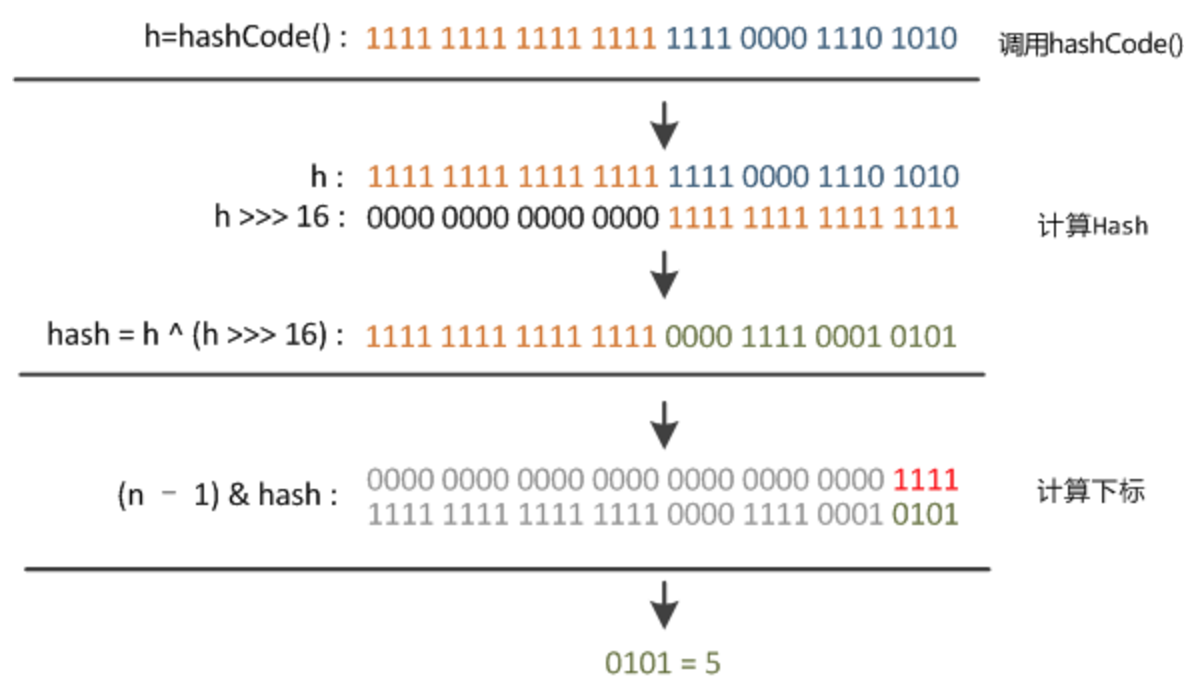static int hash(int h) {
// This function ensures that hashCodes that differ only by
// constant multiples at each bit position have a bounded
// number of collisions (approximately 8 at default load factor).
h ^= (h >>> 20) ^ (h >>> 12);
return h ^ (h >>> 7) ^ (h >>> 4);
}


//将m的所有元素存入本HashMap实例中
final void putMapEntries(Map<? extends K, ? extends V> m, boolean evict) {
int s = m.size();
if (s > 0) {
// 判断table是否已经初始化
if (table == null) { // pre-size
// 未初始化，s为m的实际元素个数
float ft = ((float)s / loadFactor) + 1.0F;
int t = ((ft < (float)MAXIMUM_CAPACITY) ?
(int)ft : MAXIMUM_CAPACITY);
// 计算得到的t大于阈值，则初始化阈值
if (t > threshold)
threshold = tableSizeFor(t);
}
// 已初始化，并且m元素个数大于阈值，进行扩容处理
else if (s > threshold)
resize();
// 将m中的所有元素添加至HashMap中
for (Map.Entry<? extends K, ? extends V> e : m.entrySet()) {
K key = e.getKey();
V value = e.getValue();
putVal(hash(key), key, value, false, evict);
}
}
}


### put()方法

putVal方法执行流程图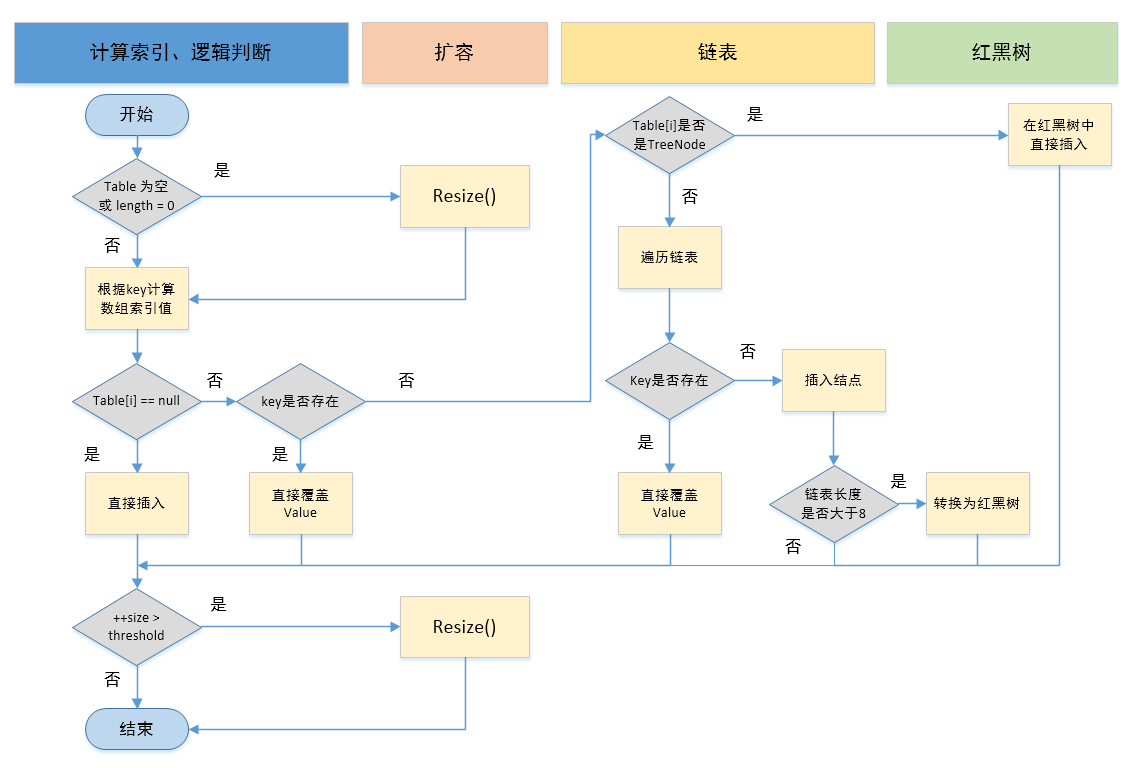public V put(K key, V value) {
return putVal(hash(key), key, value, false, true);
}

static final int hash(Object key) {
int h;
return (key == null) ? 0 : (h = key.hashCode()) ^ (h >>> 16);
}

//实现Map.put和相关方法
final V putVal(int hash, K key, V value, boolean onlyIfAbsent,
boolean evict) {
Node<K,V>[] tab; Node<K,V> p; int n, i;
// 步骤①：tab为空则创建
// table未初始化或者长度为0，进行扩容
if ((tab = table) == null || (n = tab.length) == 0)
n = (tab = resize()).length;
// 步骤②：计算index，并对null做处理
// (n - 1) & hash 确定元素存放在哪个桶中，桶为空，新生成结点放入桶中(此时，这个结点是放在数组中)
if ((p = tab[i = (n - 1) & hash]) == null)
tab[i] = newNode(hash, key, value, null);
// 桶中已经存在元素
else {
Node<K,V> e; K k;
// 步骤③：节点key存在，直接覆盖value
// 比较桶中第一个元素(数组中的结点)的hash值相等，key相等
if (p.hash == hash &&
((k = p.key) == key || (key != null && key.equals(k))))
// 将第一个元素赋值给e，用e来记录
e = p;
// 步骤④：判断该链为红黑树
// hash值不相等，即key不相等；为红黑树结点
// 如果当前元素类型为TreeNode，表示为红黑树，putTreeVal返回待存放的node, e可能为null
else if (p instanceof TreeNode)
// 放入树中
e = ((TreeNode<K,V>)p).putTreeVal(this, tab, hash, key, value);
// 步骤⑤：该链为链表
// 为链表结点
else {
// 在链表最末插入结点
for (int binCount = 0; ; ++binCount) {
// 到达链表的尾部

//判断该链表尾部指针是不是空的
if ((e = p.next) == null) {
// 在尾部插入新结点
p.next = newNode(hash, key, value, null);
//判断链表的长度是否达到转化红黑树的临界值，临界值为8
if (binCount >= TREEIFY_THRESHOLD - 1) // -1 for 1st
//链表结构转树形结构
treeifyBin(tab, hash);
// 跳出循环
break;
}
// 判断链表中结点的key值与插入的元素的key值是否相等
if (e.hash == hash &&
((k = e.key) == key || (key != null && key.equals(k))))
// 相等，跳出循环
break;
// 用于遍历桶中的链表，与前面的e = p.next组合，可以遍历链表
p = e;
}
}
//判断当前的key已经存在的情况下，再来一个相同的hash值、key值时，返回新来的value这个值
if (e != null) {
// 记录e的value
V oldValue = e.value;
// onlyIfAbsent为false或者旧值为null
if (!onlyIfAbsent || oldValue == null)
//用新值替换旧值
e.value = value;
// 访问后回调
afterNodeAccess(e);
// 返回旧值
return oldValue;
}
}
// 结构性修改
++modCount;
// 步骤⑥：超过最大容量就扩容
// 实际大小大于阈值则扩容
if (++size > threshold)
resize();
// 插入后回调
afterNodeInsertion(evict);
return null;
}


①.判断键值对数组table[i]是否为空或为null，否则执行resize()进行扩容；

②.根据键值key计算hash值得到插入的数组索引i，如果table[i]==null，直接新建节点添加，转向⑥，如果table[i]不为空，转向③；

③.判断table[i]的首个元素是否和key一样，如果相同直接覆盖value，否则转向④，这里的相同指的是hashCode以及equals；

④.判断table[i] 是否为treeNode，即table[i] 是否是红黑树，如果是红黑树，则直接在树中插入键值对，否则转向⑤；

⑤.遍历table[i]，判断链表长度是否大于8，大于8的话把链表转换为红黑树，在红黑树中执行插入操作，否则进行链表的插入操作；遍历过程中若发现key已经存在直接覆盖value即可；

⑥.插入成功后，判断实际存在的键值对数量size是否超多了最大容量threshold，如果超过，进行扩容。

### resize()方法

①.在jdk1.8中，resize方法是在hashmap中的键值对大于阀值时或者初始化时，就调用resize方法进行扩容；

②.每次扩展的时候，都是扩展2倍；

③.扩展后Node对象的位置要么在原位置，要么移动到原偏移量两倍的位置。

final Node<K,V>[] resize() {
Node<K,V>[] oldTab = table;//oldTab指向hash桶数组
int oldCap = (oldTab == null) ? 0 : oldTab.length;
int oldThr = threshold;
int newCap, newThr = 0;
if (oldCap > 0) {//如果oldCap不为空的话，就是hash桶数组不为空
if (oldCap >= MAXIMUM_CAPACITY) {//如果大于最大容量了，就赋值为整数最大的阀值
threshold = Integer.MAX_VALUE;
return oldTab;//返回
}//如果当前hash桶数组的长度在扩容后仍然小于最大容量 并且oldCap大于默认值16
else if ((newCap = oldCap << 1) < MAXIMUM_CAPACITY &&
oldCap >= DEFAULT_INITIAL_CAPACITY)
newThr = oldThr << 1; // double threshold 双倍扩容阀值threshold
}
// 旧的容量为0，但threshold大于零，代表有参构造有cap传入，threshold已经被初始化成最小2的n次幂
// 直接将该值赋给新的容量
else if (oldThr > 0) // initial capacity was placed in threshold
newCap = oldThr;
// 无参构造创建的map，给出默认容量和threshold 16, 16*0.75
else {               // zero initial threshold signifies using defaults
newCap = DEFAULT_INITIAL_CAPACITY;
}
// 新的threshold = 新的cap * 0.75
if (newThr == 0) {
float ft = (float)newCap * loadFactor;
newThr = (newCap < MAXIMUM_CAPACITY && ft < (float)MAXIMUM_CAPACITY ?
(int)ft : Integer.MAX_VALUE);
}
threshold = newThr;
// 计算出新的数组长度后赋给当前成员变量table
@SuppressWarnings({"rawtypes","unchecked"})
Node<K,V>[] newTab = (Node<K,V>[])new Node[newCap];//新建hash桶数组
table = newTab;//将新数组的值复制给旧的hash桶数组
// 如果原先的数组没有初始化，那么resize的初始化工作到此结束，否则进入扩容元素重排逻辑，使其均匀的分散
if (oldTab != null) {
// 遍历新数组的所有桶下标
for (int j = 0; j < oldCap; ++j) {
Node<K,V> e;
if ((e = oldTab[j]) != null) {
// 旧数组的桶下标赋给临时变量e，并且解除旧数组中的引用，否则就数组无法被GC回收
oldTab[j] = null;
// 如果e.next==null，代表桶中就一个元素，不存在链表或者红黑树
if (e.next == null)
// 用同样的hash映射算法把该元素加入新的数组
newTab[e.hash & (newCap - 1)] = e;
// 如果e是TreeNode并且e.next!=null，那么处理树中元素的重排
else if (e instanceof TreeNode)
((TreeNode<K,V>)e).split(this, newTab, j, oldCap);
// e是链表的头并且e.next!=null，那么处理链表中元素重排
else { // preserve order
Node<K,V> loHead = null, loTail = null;
Node<K,V> hiHead = null, hiTail = null;
Node<K,V> next;
// 遍历链表
do {
next = e.next;
if ((e.hash & oldCap) == 0) {
if (loTail == null)
// 代表下标保持不变的链表的头元素
else
// loTail.next指向当前e
loTail.next = e;
// loTail指向当前的元素e
loTail = e;
}
else {
if (hiTail == null)
else
hiTail.next = e;
hiTail = e;
}
} while ((e = next) != null);
// 遍历结束, 将tail指向null，并把链表头放入新数组的相应下标，形成新的映射。
if (loTail != null) {
loTail.next = null;
}
if (hiTail != null) {
hiTail.next = null;
}
}
}
}
}
return newTab;
}


### treeifyBin()方法

​ 1、判断桶是否初始化、或者判断桶中的元素个数是否达到MIN_TREEIFY_CAPACITY阈值，没有的话则去进行初始化或者扩容

​ 2、若不符合上述条件，则会对其进行树形化，首先会先去遍历桶中链表的元素，并创建相同的树节点，接着会根据桶的第一个元素而去创建树的头结点，并以此建立联系

final void treeifyBin(Node<K,V>[] tab, int hash) {
int n, index; Node<K,V> e;
if (tab == null || (n = tab.length) < MIN_TREEIFY_CAPACITY)
resize();
//开始树形化
else if ((e = tab[index = (n - 1) & hash]) != null) {
TreeNode<K,V> hd = null, tl = null;
//对桶Node中的链表元素进行循环，从链表的头节点开始将链表的头元素改为树的头节点
do {
TreeNode<K,V> p = replacementTreeNode(e, null);
if (tl == null)
hd = p;
else {
//树的头节点不为空时
p.prev = tl;
tl.next = p;
}
tl = p;
} while ((e = e.next) != null);
//将桶中的元素与树的头节点进行连接
if ((tab[index] = hd) != null)
hd.treeify(tab);
}
}


### get()方法

public V get(Object key) {
Node<k,v> e;
return (e = getNode(hash(key), key)) == null ? null : e.value;
}

final Node<K,V> getNode(int hash, Object key) {
Node<K,V>[] tab; Node<K,V> first, e; int n; K k;
// table已经初始化，长度大于0，根据hash寻找table中的项也不为空
if ((tab = table) != null && (n = tab.length) > 0 &&
(first = tab[(n - 1) & hash]) != null) {
// 桶中第一项(数组元素)相等
if (first.hash == hash && // always check first node
((k = first.key) == key || (key != null && key.equals(k))))
return first;
// 桶中不止一个结点
if ((e = first.next) != null) {
// 为红黑树结点
if (first instanceof TreeNode)
// 在红黑树中查找
return ((TreeNode<K,V>)first).getTreeNode(hash, key);
// 否则，在链表中查找
do {
if (e.hash == hash &&
((k = e.key) == key || (key != null && key.equals(k))))
return e;
} while ((e = e.next) != null);
}
}
return null;
}


### remove()方法

/**
* 从HashMap中删除掉指定key对应的键值对，并返回被删除的键值对的值
* 如果返回空，说明key可能不存在，也可能key对应的值就是null
* 如果想确定到底key是否存在可以使用containsKey方法
*/
public V remove(Object key) {
Node<K,V> e; // 定义一个节点变量，用来存储要被删除的节点（键值对）
return (e = removeNode(hash(key), key, null, false, true)) == null ?
null : e.value; // 调用removeNode方法
}


/**
* 方法为final，不可被覆写，子类可以通过实现afterNodeRemoval方法来增加自己的处理逻辑（解析中有描述）
*
* @param hash key的hash值，该值是通过hash(key)获取到的
* @param key 要删除的键值对的key
* @param value 要删除的键值对的value，该值是否作为删除的条件取决于matchValue是否为true
* @param matchValue 如果为true，则当key对应的键值对的值equals(value)为true时才删除；否则不关心value的值
* @param movable 删除后是否移动节点，如果为false，则不移动
* @return 返回被删除的节点对象，如果没有删除任何节点则返回null
*/
final Node<K,V> removeNode(int hash, Object key, Object value,
boolean matchValue, boolean movable) {
Node<K,V>[] tab; Node<K,V> p; int n, index; // 声明节点数组、当前节点、数组长度、索引值
/*
* 如果 节点数组tab不为空、数组长度n大于0、根据hash定位到的节点对象p（该节点为 树的根节点 或 链表的首节点）不为空
* 需要从该节点p向下遍历，找到那个和key匹配的节点对象
*/
if ((tab = table) != null && (n = tab.length) > 0 &&
(p = tab[index = (n - 1) & hash]) != null) {
Node<K,V> node = null, e; K k; V v; // 定义要返回的节点对象，声明一个临时节点变量、键变量、值变量

// 如果当前节点的键和key相等，那么当前节点就是要删除的节点，赋值给node
if (p.hash == hash &&
((k = p.key) == key || (key != null && key.equals(k))))
node = p;

/*
* 到这一步说明首节点没有匹配上，那么检查下是否有next节点
* 如果没有next节点，就说明该节点所在位置上没有发生hash碰撞, 就一个节点并且还没匹配上，也就没得删了，最终也就返回null了
* 如果存在next节点，就说明该数组位置上发生了hash碰撞，此时可能存在一个链表，也可能是一颗红黑树
*/
else if ((e = p.next) != null) {
// 如果当前节点是TreeNode类型，说明已经是一个红黑树，那么调用getTreeNode方法从树结构中查找满足条件的节点
if (p instanceof TreeNode)
node = ((TreeNode<K,V>)p).getTreeNode(hash, key);
// 如果不是树节点，那么就是一个链表，只需要从头到尾逐个节点比对即可
else {
do {
// 如果e节点的键是否和key相等，e节点就是要删除的节点，赋值给node变量，调出循环
if (e.hash == hash &&
((k = e.key) == key ||
(key != null && key.equals(k)))) {
node = e;
break;
}

// 走到这里，说明e也没有匹配上
p = e; // 把当前节点p指向e，这一步是让p存储的永远下一次循环里e的父节点，如果下一次e匹配上了，那么p就是node的父节点
} while ((e = e.next) != null); // 如果e存在下一个节点，那么继续去匹配下一个节点。直到匹配到某个节点跳出 或者 遍历完链表所有节点
}
}

/*
* 如果node不为空，说明根据key匹配到了要删除的节点
* 如果不需要对比value值  或者  需要对比value值但是value值也相等
* 那么就可以删除该node节点了
*/
if (node != null && (!matchValue || (v = node.value) == value ||
(value != null && value.equals(v)))) {
if (node instanceof TreeNode) // 如果该节点是个TreeNode对象，说明此节点存在于红黑树结构中，调用removeTreeNode方法（该方法单独解析）移除该节点
((TreeNode<K,V>)node).removeTreeNode(this, tab, movable);
else if (node == p) // 如果该节点不是TreeNode对象，node == p 的意思是该node节点就是首节点
tab[index] = node.next; // 由于删除的是首节点，那么直接将节点数组对应位置指向到第二个节点即可
else // 如果node节点不是首节点，此时p是node的父节点，由于要删除node，所有只需要把p的下一个节点指向到node的下一个节点即可把node从链表中删除了
p.next = node.next;
++modCount; // HashMap的修改次数递增
--size; // HashMap的元素个数递减
afterNodeRemoval(node); // 调用afterNodeRemoval方法，该方法HashMap没有任何实现逻辑，目的是为了让子类根据需要自行覆写
return node;
}
}
return null;
}


### 遍历

HashMap的四种遍历方式

//HashMap的四种遍历方式
public static void main(String[] args) {
Map<String, String> map = new HashMap<String, String>();
map.put("1", "value1");
map.put("2", "value2");
map.put("3", "value3");
map.put("4", "value4");

//第一种   通过Map.entrySet遍历,推荐使用,尤其是容量大时
System.out.println("通过Map.entrySet遍历key和value: ");
for (Map.Entry<String, String> entry : map.entrySet()) {
System.out.println("Key: " + entry.getKey() + " - Value: " + entry.getValue());
}

//第二种   通过Map.entrySet使用iterator遍历
System.out.println("\n通过Map.entrySet使用iterator遍历key和value: ");
Iterator map1it = map.entrySet().iterator();
while (map1it.hasNext()) {
Map.Entry<String, String> entry = (Map.Entry<String, String>) map1it.next();
System.out.println("Key: " + entry.getKey() + " - Value: " + entry.getValue());
}

//第三种   通过Map.keySet遍历,二次取值
System.out.println("\n通过Map.keySet遍历key和value: ");
for (String key : map.keySet()) {
System.out.println("Key: " + key + " - Value: " + map.get(key));
}

//第四种   通过Map.values()遍历
System.out.println("\n通过Map.values()遍历所有的value,但不能遍历key: ");
for (String v : map.values()) {
System.out.println("The value is " + v);
}
}

03-1510万+
06-056万+01-066万+
09-031万+
04-245万+
10-126万+
05-061893
09-138632
01-076万+
11-0225万+